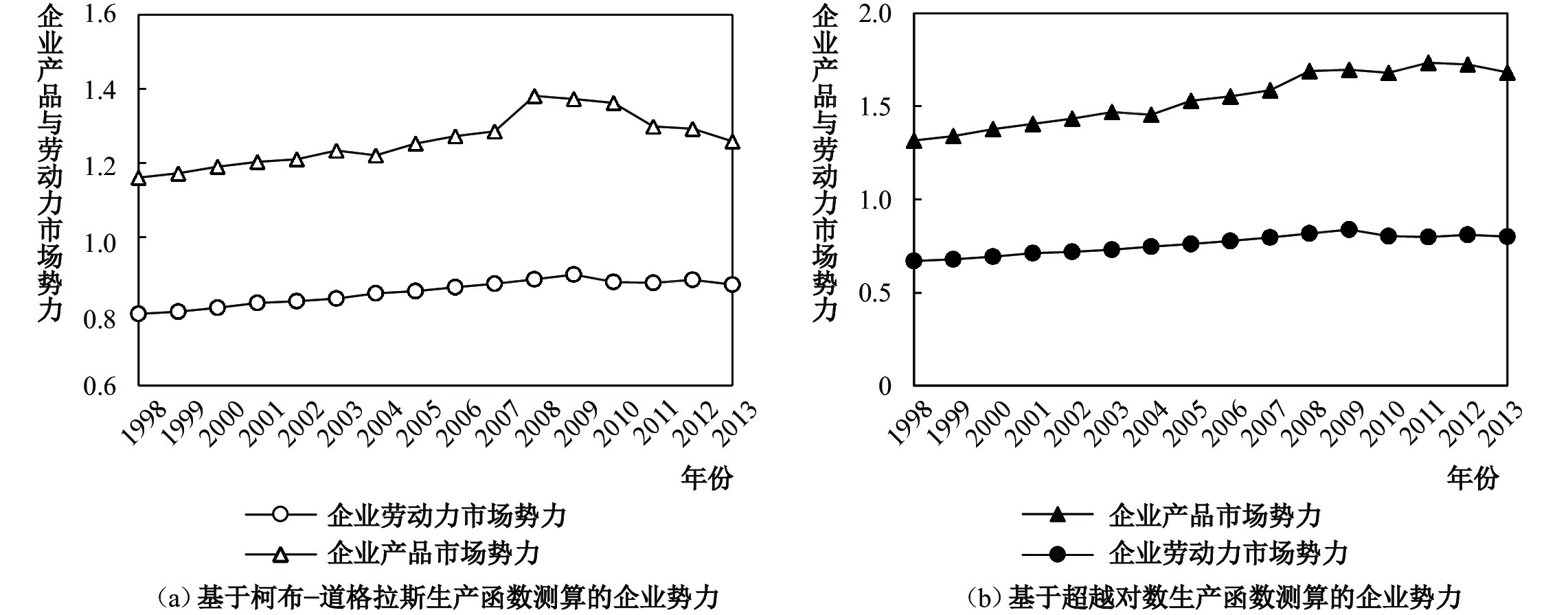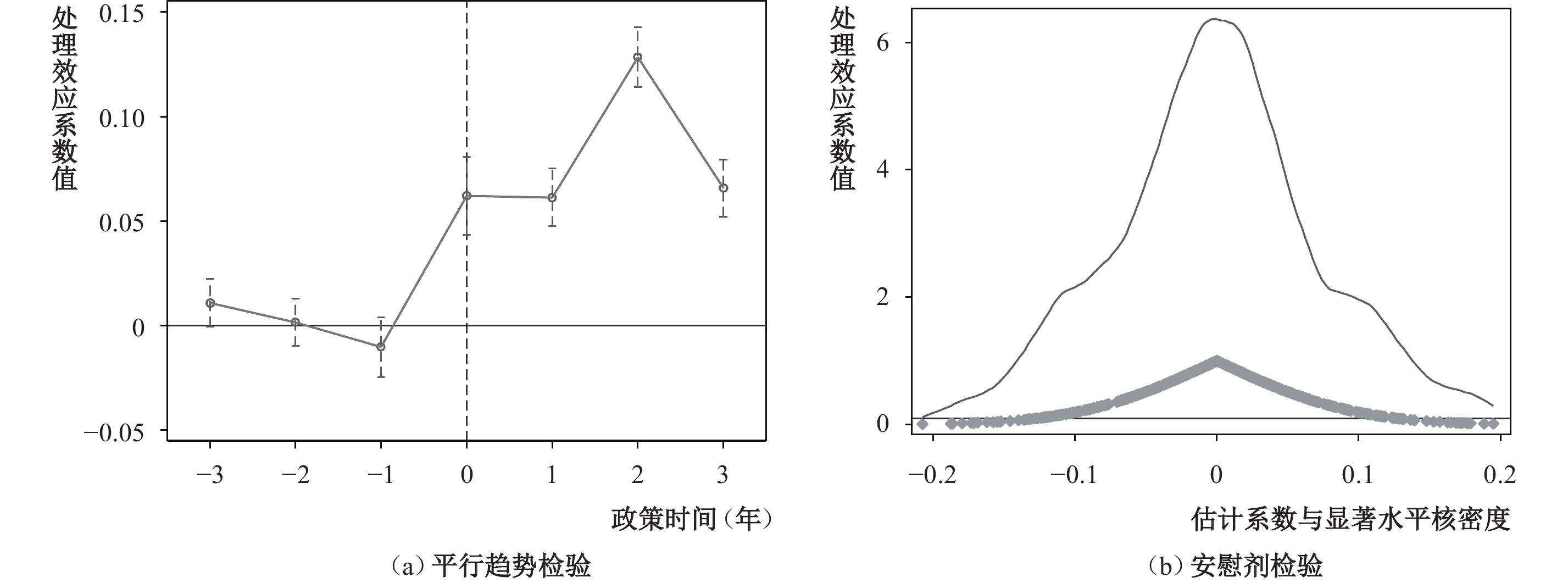﻿ 市场势力关联的收入分配效应：关联机理识别与竞争政策优化《财经研究》
2023第49卷第7期

1. 浙江工商大学 经济学院，浙江 杭州 310018;
2. 中国社会科学院 工业经济研究所，北京 100006;
3. 东北大学 工商管理学院，辽宁 沈阳 110167

Income Distribution Effect of Market Power Association: Correlation Mechanism Identification and Competition Policy Optimization
Zhao Weiguang1, Li Wei2, Li Kai3
1. School of Economics, Zhejiang Gongshang University, Hangzhou 310018, China;
2. Institute of Industrial Economics, Chinese Academy of Social Sciences, Beijing 100006, China;
Summary: This paper introduces market power into the issue of income distribution. Based on a combined sample consisting of industrial firm data, antitrust administrative and civil litigation data, and minimum wage data, a “firm-employee” private bargaining model is constructed to empirically study the wage determination mechanism under the scenario of imperfect competition. By measuring the market power of both products and labor, it is found that there is a “bilateral market power association” between products and labor, and firms can adjust the allocation of bilateral market power according to changes in the competitive environment. In this scenario, the behavior of firms expanding their power to the labor market not only reduces the rent-sharing ability of employees, but also to some extent widens the wage gap between skilled workers. The conclusions are as follows: (1) From 1998 to 2013, the market power of products showed a trend of first rising and then falling, while the market power of labor showed a trend of first falling and then rising, indicating a statistical substitution relationship between the market power of products and labor. (2) In this scenario, although the decline of the market power of products is beneficial to increasing the rent-sharing ability of employees, the behavior of firms expanding their power to the labor market caused by the decline of the market power of products will widen the income gap between different skilled workers. (3) From the decomposition results of the market power of products and labor market, the improvement of firms’ own production efficiency explains most of the increase in market power, but the income distribution effect generated by firms’ strategic use of their market power requires attention. (4) Counterfactual policy tests show that promoting the elimination of the market power of both products and labor will not only increase the rent-sharing ability of employees by about 10.57%, but also reduce the wage gap by about 12.15%. The marginal contributions are that: (1) This paper constructs a computable partial equilibrium model that reveals the internal influence mechanism of market power on employees’ rent-sharing and wage gap. The framework is based on the “firm- employee” private bargaining model, which better fits the Chinese economic reality. (2) This paper develops a method for quantitatively identifying the market power of products and labor under the private bargaining mechanism. This identification method only requires output data and employee wage data at the firm level, thus having generality, which expands the literature research on identifying market power. (3) The advantage of using a partial equilibrium model is that counterfactual policy simulations can be conducted to evaluate the impact of different policy combinations on employees’ rent-sharing elasticity and skilled wage gaps, providing empirical references for government decision-making.
Key words: bilateral market power association    rent-share    skill wage gap    competition policy

（一）劳动者的决策。假定在市场中有J个企业，企业在具体生产过程中需要雇佣两种类型的劳动者，低技能劳动者I和高技能劳动者H。每个企业j∈[1，…，J]对不同技能的劳动者支付工资（wIj，wHj）。由于企业具有异质性，员工对不同企业提供的工作岗位具有异质性偏好。属于技能组S∈[IH]的劳动者i选择在企业j工作的效用可以表示为：

 ${U}_{iSj}={\beta }_{S}\mathrm{L}\mathrm{n}\left({w}_{Sj}-{w}_{aj}\right)+{\delta }_{Sj}+{\varepsilon }_{iSj}$ (1)

 $P\left({{\mathrm{argmax}}\left\{{U}_{iSk}\right\}}_{k\in \left[1,\dots ,J\right]}=j\right)=\frac{\mathrm{e}\mathrm{x}\mathrm{p}({\beta }_{S}\mathrm{L}\mathrm{n}\left({w}_{Sj}-{w}_{aj}\right)+{\delta }_{Sj})}{{\sum }_{k=1}^{J}\mathrm{e}\mathrm{x}\mathrm{p}({\beta }_{s}\mathrm{L}\mathrm{n}\left({w}_{Sk}-{w}_{ak}\right)+{\delta }_{Sk})}$ (2)

 $P\left({{\mathrm{argmax}}\left\{{U}_{iSk}\right\}}_{k\in \left[1,\dots ,J\right]}=j\right)={\lambda }_{S}\mathrm{exp}\left[{\beta }_{S}\mathrm{L}\mathrm{n}\left({w}_{Sj}-{w}_{aj}\right)+{\delta }_{Sj}\right]$ (3)

 $\mathrm{L}\mathrm{n}{I}_{j}\left({w}_{Ij}\right)=\mathrm{L}\mathrm{n}\left({\lambda }_{I}\right)+{\beta }_{I}\mathrm{L}\mathrm{n}\left({w}_{Sj}-{w}_{aj}\right)+{\delta }_{Ij}$ (4)
 $\mathrm{L}\mathrm{n}{H}_{j}\left({w}_{Hj}\right)=\mathrm{L}\mathrm{n}\left({\lambda }_{H}\right)+{\beta }_{H}\mathrm{L}\mathrm{n}\left({w}_{Sj}-{w}_{aj}\right)+{\delta }_{Hj}$ (5)

（二）企业的决策。与Card等（2018）构建的局部均衡框架不同，本部分构建了一个企业与员工进行私人议价的理论模型，揭示不完全竞争情境下的工资决定机制。企业在生产过程中的总利润函数可以表示为：

 ${\pi }_{j}={P}_{j}\left({Q}_{j}\right){Q}_{j}-r{K}_{j}-{\sum }_{i\in {L}_{i}}{w}_{Sj}\left({L}_{Sij}\right){L}_{Sij}$ (6)

 ${\pi }_{j}\left(-i\right)={R}_{j}\left(-i\right)-{\sum }_{i\in {L}_{i}\backslash i}{w}_{Sj}\left({L}_{Sj}\right){L}_{Sj}$ (7)

 ${w}_{Sj}{L}_{Sj}={\mathrm{min}}\Biggr\{ \underbrace{\dfrac{{\alpha }_{Sj}}{1 - {\alpha }_{Sj}}\left[{R}_{j} - {\sum }_{i\in {L}_{i}}{w}_{Sj}\left({L}_{Sj}\right){L}_{Sj}\right] + {w}_{aSj}\left({L}_{Sj}\right){L}_{Sj}}_{(a)} , \; \underbrace{{\alpha }_{Sj}\left[{R}_{j}-{R}_{j}\left(-i\right)\right]+\left(1 - {\alpha }_{Sj}\right){w}_{aSj}\left({L}_{Sj}\right){L}_{Sj}}_{(b)}\Biggr\}$ (8)

 ${\pi }_{j}\left({w}_{Sj}{L}_{Sj},{{w}_{Sk}^{*}{L}_{Sk}^{*}}\right)=\left(1-{\alpha }_{Sj}\right){R}_{j}+{\alpha }_{Sj}{R}_{j}\left(-i\right)- \sum _{k\in {L}_{i}\backslash i}{w}_{k}^{*}{L}_{k}^{*}-\left(1-{\alpha }_{Sj}\right){w}_{Sj}\left({L}_{Sj}\right){L}_{Sj}$ (9)

 $\frac{\partial {\pi }_{j}}{\partial {L}_{Sj}}=\frac{\partial {P}_{j}}{\partial {Q}_{j}}\frac{\partial {Q}_{j}}{\partial {L}_{Sj}}{Q}_{j}+{P}_{j}\frac{\partial {Q}_{j}}{\partial {L}_{Sj}}-{w}_{aSj}-{L}_{Sj}\frac{\partial {w}_{aSj}}{\partial {L}_{Sj}}=0$ (10)

 $\frac{{w}_{aSj}}{{w}_{Sj}}\left(1+\frac{1}{{\varepsilon}_{Sj}^{L}}\right)=\frac{{\theta }_{Sj}^{L}}{{\alpha }_{Sj}^{L}}\left|1+\frac{1}{{\varepsilon}_{j}^{p}}\right|$ (11)

 ${MP}_{j}=\frac{{MU}_{j}}{{MD}_{Sj}}=\frac{{\theta }_{Sj}^{L}}{{\alpha }_{Sj}^{L}}\frac{{w}_{Sj}}{{w}_{aSj}}$ (12)

（三）不完全竞争市场环境下的工资决定机制。根据Card等（2018）的理论模型，员工议价能力可以进一步表示为劳动供给弹性的函数，即 ${\alpha }_{Sj}={\beta }_{S}/(1+{\beta }_{S})$ ，其中 ${\beta }_{s}$ 为式（4）与式（5）的回归系数。据此，可将上述私人议价模式下的工资方程进一步表示为：

 ${w}_{Sj}=\frac{1}{1+{\beta }_{S}}{w}_{aSj}+\frac{{\beta }_{S}}{1+{\beta }_{S}}\frac{\left[{R}_{j}-{R}_{j}\left(-i\right)\right]}{{L}_{Sj}}$ (13)

 ${\mathrm{L}\mathrm{n}w}_{Sj}=\mathrm{L}\mathrm{n}\frac{1}{1+{\beta }_{S}}{w}_{aSj}+\mathrm{L}\mathrm{n}\left(1+{\beta }_{S}{R}_{j}\right)$ (14)

 ${\xi }_{Sj}=\frac{\partial {\mathrm{L}\mathrm{n}w}_{Sj}}{\partial \mathrm{L}\mathrm{n}{v}_{j}}=\frac{{\beta }_{S}{R}_{j}}{1+{\beta }_{S}{R}_{j}}$ (15)

 $\mathrm{L}\mathrm{n}\frac{{w}_{Hj}}{{w}_{Ij}}=\mathrm{L}\mathrm{n}\frac{1+{\beta }_{I}}{1+{\beta }_{H}}+\mathrm{L}\mathrm{n}\left(\frac{1+{\beta }_{H}{R}_{j}}{1+{\beta }_{I}{R}_{j}}\right)$ (16)

（一）市场势力测度。式（12）衡量的就是企业在产品与劳动力市场中的联合市场势力 ${MP}_{j}$ ，等于劳动投入的产出弹性 ${\theta }_{jt}^{L}$ 与劳动收入占企业总产值的份额 ${\alpha }_{jt}^{L}$ 之比，乘以平均工资wSj与保留工资waSj之比。其中 ${\alpha }_{jt}^{L}$ 可以根据工业企业数据库直接计算， ${\theta }_{jt}^{L}$ 可以通过估计企业层面的生产函数的系数值来获得。本文采用Wooldridge半参数法估算具有柯布–道格拉斯生产技术的生产函数。 2 其关键在于如何测算企业产品市场势力与劳动力市场势力。实际上，式（11）与式（12）已经给出了私人议价模式下的企业产品与劳动力市场势力测算的表达式：

 ${MU}_{jt}=\frac{{p}_{jt}}{{mc}_{jt}}=\frac{\left|{\varepsilon}_{jt}^{p}\right|}{\left|{\varepsilon}_{jt}^{p}\right|-1}$ (17)
 ${MD}_{jt}=\frac{{w}_{jt}}{{MRPL}_{jt}}=\frac{\left|{\varepsilon}_{jt}^{L}\right|}{\left|{\varepsilon}_{jt}^{L}\right|+1}$ (18)

（二）实证模型设计。本文实证模型包括如下两个部分：

1. 企业市场势力作用于员工租金分享能力的实证识别。为了避免企业利润与工资间内生性问题引发的识别偏差，本文用第三部分构建的私人议价下的企业势力作用于租金分享的可量化局部均衡模型对员工租金分享能力进行测算。具体而言，首先，根据私人议价下的企业市场势力识别方法，对中国企业在产品与劳动力市场中的市场势力进行测算；其次，将测算的企业产品与劳动力市场势力代入到（15）式，对员工租金分享能力进行测算；最后，用反事实分析消除企业市场势力带来的潜在租金分享提高程度。

2. 企业市场势力作用于员工技能工资差距的实证识别。本部分以 2008 年《反垄断法》实施构建准自然实验，结合中国微观企业数据，采用双重差分模型，系统考察产品与劳动力市场势力对员工租金分享弹性以及技能工资差距的影响。反垄断法实施为识别企业市场势力作用于员工工资决定机制提供了一个准自然实验窗口，反垄断法作为产品市场的外生冲击，仅对产品市场的垄断行为进行规制，并不涉及劳动力市场（王彦超和蒋亚含，2020）。一方面，如果确实存在企业向劳动力市场扩展势力现象，那么表征反垄断法实施的政策处理效应回归系数应在统计上显著；另一方面，采用准自然实验方法使劳动力市场因素不能反向影响产品市场，可以有效避免互为因果引发的内生性问题。具体而言，构建如下DID识别系统：

 ${MU}_{jt}={\alpha }_{0}+{\alpha }_{1}{AAC}_{jt}+{X}_{jt}^{'}\delta +{\omega }_{h}+{\omega }_{t}+{\omega }_{p}+{\varepsilon }_{jt}$ (19)

 $\mathrm{L}\mathrm{n}{gap}_{jt}={\alpha }_{0}+{\alpha }_{1}\Delta {MU}_{jt}+{\alpha }_{2}{AAC}_{jt}\times \Delta {MU}_{jt}+{X}_{jt}^{'}+{\omega }_{h}+{\omega }_{t}+{\omega }_{p}+{\varepsilon }_{jt}$ (20)

（三）数据说明。本文数据包括以下三部分：一是1998—2013年中国工业企业数据库的企业数据。参考已有文献对行业代码、企业规模口径、缺失值、明显统计错误及不符合会计准则样本进行处理。二是2008—2013年间中国反垄断执法机构发布的全部行政处罚和垄断民事诉讼决定报告等反垄断执法信息数据。其中，行政处罚数据来源于中国反垄断执法机构官网发布的全部行政处罚决定书、公告和案件新闻；民事诉讼数据来源于中国裁判文书网。将其按照企业名称、法人代表和地址等信息与工业企业数据库匹配。三是从《中国统计年鉴》中手工收集了各地区的农村个人劳动收入数据（l_income）、最低工资数据（m_income）和教育统计数据。工业企业数据仅在2004年给出了企业员工学历构成信息。本文将1998—2013年的各企业技能与非技能员工比例固定在2004年，并按照不同省份的各阶段教育人数增长情况进行调整，用以计算企业内部低技能与高技能员工面临的企业劳动力市场势力。

（一）私人议价模式下的企业产品与劳动力市场势力测算。私人议价模式下的企业产品与劳动力市场势力测算结果如图1所示。 4 从中可以看出，企业不仅在产品市场获取垄断租金，也会通过压低员工收入的方式，进一步获取劳动租金，意味着企业在产品与劳动力市场都具有一定的市场势力，这与简泽等（2016）的分析是一致的。平均而言，企业在产品市场获取高于边际成本66.13%的价格加成，在劳动力市场支付低于劳动边际产品收益24.31%的工资。从时间趋势来看，企业产品市场势力呈现出先上升后降低的趋势，由1998年的1.16上升到2008年的1.38，随后下降到2013年的1.25。与之对应的是，企业劳动力市场势力则呈现出先下降后上升的趋势，工资占劳动收益比率由1998年的79.34%提高到2009年的89.94%，随后下降到2013年的87.18%。以上特征事实表明，企业在产品与劳动力市场中的市场势力呈现出统计上的替代关系。这种关联关系意味着企业可以通过减弱产品市场势力同时增加劳动力市场势力的方式来维持企业整体市场势力不变（赵伟光等，2022）。图 1 1998—2013 年中国制造企业产品与劳动力市场势力

（二）不完全竞争视角下的员工租金分享能力测算。本文基于可量化局部均衡模型推导出的式（15），结合上述计算的企业产品与劳动力市场势力，测算了1998—2013年间的企业员工租金分享能力，结果如表1所示。总体来看，1998—2009年间，随着企业产品市场势力的增强以及劳动力市场势力的减弱，员工租金分享能力由1998年的0.09提高到2009年的0.18。这意味着平均而言企业增加值每提高1%，会使员工工资提高约18%。然而，在2009年之后，随着企业产品市场势力的下降以及劳动力市场势力的增强，员工租金分享能力由2009年的0.18下降到2013年的0.15，这与周维和齐建国（2014）的结论一致，说明中国企业员工租金分享能力呈现出先上升后下降的变动趋势。与之不同的是，本研究发现，企业在产品与劳动力市场中的市场势力是影响员工租金分享能力趋势变动的重要因素。企业垄断势力不仅影响市场运行效率，还具有潜在的收入分配效应。采用可量化局部均衡模型的优势还在于可以进行国际比较。Card等（2018）发现，发达国家租金分享弹性均值约为0.16。本文测算的中国制造企业租金分享弹性为0.166，可以看出，就国际比较而言，中国企业不存在企业利润侵蚀员工工资现象。

 年份 MU MD rentshare 年份 MU MD rentshare 1998 1.160 0.793 0.091 2006 1.272 0.865 0.140 1999 1.173 0.799 0.095 2007 1.286 0.875 0.151 2000 1.190 0.809 0.101 2008 1.381 0.886 0.168 2001 1.203 0.823 0.111 2009 1.373 0.899 0.184 2002 1.210 0.827 0.112 2010 1.362 0.879 0.184 2003 1.234 0.834 0.119 2011 1.298 0.877 0.159 2004 1.220 0.848 0.124 2012 1.292 0.884 0.165 2005 1.253 0.855 0.134 2013 1.258 0.872 0.151 注：企业租金分享能力rentshare基于式（15）测算，企业产品市场势力MU基于式（17）测算，企业劳动力市场势力MD基于式（18）测算。

（三）“产品—劳动力”市场势力关联与员工技能工资差距识别。表2列（1）报告了式（19）估计结果，post系数回归结果表明，反垄断处罚确实使得涉事企业所在省份行业的产品市场势力呈现出下降趋势。列（2）给出了式（20）回归结果，为了控制可能影响技能工资差距的其他因素，加入企业规模和企业间技术距离等控制变量（ ${X}_{jt}^{'}$ ）。同时控制省份、行业和年份固定效应对估计结果的影响。结果表明，处理效应did×ΔMU估计系数显著为0.05，说明反垄断处罚造成的涉事省份行业产品市场势力下降，使劳动力市场中员工技能工资差距扩大了5%。这初步证明了企业向劳动力市场的市场势力扩展行为确实会使员工技能工资差距扩大。为了进一步证明市场势力扩展现象的存在。列（3）用企业劳动力市场势力作为被解释变量，对式（20）进行重新回归，结果表明，反垄断处罚引发的企业产品市场势力下降，使企业劳动力市场势力呈现出增长趋势。进一步地，列（4）将处理效应did×ΔMU与企业劳动力市场势力组成交互项，对式（20）进行重新回归，结果表明，交互项系数为–0.12且在统计上显著，说明企业向劳动力市场扩展势力行为确实是导致员工技能工资差距扩大的重要因素。为了检验企业市场势力扩展行为对员工租金分享能力的影响，列（5）将租金分享能力rentshare作为被解释变量，对式（20）进行重新回归，结果表明，企业产品市场势力的减弱会使员工租金分享能力提高约0.9%。上述结论证明，在双边市场势力关联的环境中，存在贾俊雪和孙传辉（2019）提出的垄断情境下的效率与公平权衡问题。不同的是，本研究证明了双边市场势力关联是形成效率与公平权衡的重要内在机理。

 （1）MU （2）lngap （3）MD （4）lngap （5）rentshare ΔMU 0.251***（0.001） 0.250***（0.001） 0.009***（0.000） did×ΔMU 0.053***（0.004） 0.001***（0.000） post −1.117***（0.002） −0.016***（0.000） post×ΔMU×ΔMD −0.117***（0.032） 控制变量 控制 控制 控制 控制 控制 三项固定效应 控制 控制 控制 控制 控制 样本量 1 715 389 1 163 984 1 378 734 1 163 984 1 426 190 拟合优度 0.487 0.437 0.014 0.439 0.280 注：（1）***、**和*表示在1%、5%和10%水平上显著；（2）括号中为标准误，三项固定效应表示年份、省份和行业固定效应；（3）为了节省篇幅，未展示控制变量估计结果，如有需要可联系作者。下表统同。

（四）稳健性检验。 6 第一，企业向劳动力市场扩展势力引发的收入分配效应得以实现的前提是劳动力市场势力不能影响反垄断处罚，即不存在反向因果造成的内生性问题。为此，将企业是否受到反垄断处罚作为被解释变量，将企业劳动力市场势力作为解释变量，对上述前提假设进行实证检验，结果表明，企业劳动力市场势力并不影响反垄断处罚。第二，如果确实存在本文提出的双边市场势力关联效应，企业整体市场势力应该不变或者变动不大。为此，本文将企业市场势力MP作为被解释变量，替换式（20）进行重新回归。结果表明，反垄断处罚虽然削弱了企业产品市场势力，但由于存在企业向劳动力市场扩展势力的行为，使企业整体市场势力变动不大。第三，实际上，在2008年，国家还进行了其他行政性改革，这些政策可能会对工资差距以及企业在产品与劳动力市场中的势力产生影响，从而造成统计上的虚假显著性。为此，参照赵伟光等（2022）的指标设定方法，在式（20）基础上控制4万亿经济刺激计划和新《劳动合同法》政策实施对估计结果的影响。结果表明，在控制其他政策改革影响后，企业市场势力引发的收入分配效应依然存在。第四，DID检验得以成立的前提还在于处理组和控制组分组的随机性。为此，选择跨省份边界的地级市内企业样本对式（20）进行重新回归，结果显示，处理效应估计系数依然显著为正。第五，根据Card等（2018）的研究，地理和行业划分范围是影响企业劳动力市场势力指数大小的关键因素。为此，在三位数地区和行业代码基础上，对企业劳动力市场势力进行重新测算，并将新指标替换到式（20）中对结论的稳健性进行检验，结果表明，企业向劳动力市场扩展势力引发的收入分配效应依然存在。第六，为了控制产品与要素价格扭曲对市场势力识别的影响，本文用超越对数生产函数重新测算了企业产品与劳动力市场势力，并用新生成的产品市场势力TMU作为替代变量对式（20）进行回归。结果显示，即使考虑到要素价格扭曲的影响，市场势力引发的收入分配效应依然存在。

1. 平行趋势检验。以2008年为界，将2005–2007年设定为政策执行前，将2009–2012年设定为政策执行后进行平行趋势检验。平行趋势检验结果如图2（a）所示。结果表明，在反垄断法执行前，处理效应系数值接近于0且不具有统计显著性，说明在政策执行前处理组和控制组具有趋势一致性，满足趋势一致性假定。图 2 平行趋势检验与安慰剂检验

2. 安慰剂检验。为了检验DID是否满足政策干预的独立性，本研究进行了如下安慰剂检验。通过随机设定处理组和对照组的方式生成虚假政策处理效应，对式（20）进行回归并记录估计结果，再将上述实验设计循环500次。从图2（b）安慰剂检验结果可以看出，虚假政策处理效应系数值在0值附近，且P值不显著，说明满足DID政策干预的独立性假定。

（五）基于DOP的企业产品与劳动力市场势力分解。从表3显示的产品与劳动力市场势力分解结果来看，企业自身生产效率提高解释了大部分的市场势力提升。例如，就产品市场而言，企业生产率提高对势力的贡献从1998—2003年间的58.52%提升到2004—2008年间的65.89%。就劳动力市场而言，企业生产率提高对势力的贡献从1998—2003年间的90.18%提升到2004—2008年间的91.60%。这意味着企业市场势力的提高大部分是由企业自身生产效率提升引致的。具体而言，劳动力市场配置效率的提高提升了劳动边际产出价值。然而，企业向劳动力市场扩展势力的行为却使员工实际工资增长幅度并未赶上劳动边际产出价值的增长幅度，从而造成实际工资与劳动边际产出价值的差距扩大。根据产业组织理论，市场势力本身是一个中性概念，企业由于生产效率增加获取的市场势力并不是反垄断干预的重点。但是，企业向劳动力市场扩展势力形成的收入差距扩大问题则需要竞争法的介入。

 产品市场势力分解 年份 变动率 组内效应 组间效应 进入效应 退出效应 1998—2003 0.879 0.515 0.281 −0.001 0.085 2004—2008 0.669 0.441 0.214 −0.023 0.037 2009—2013 0.249 0.053 0.229 −0.059 0.028 劳动力市场势力分解 年份 变动率 组内效应 组间效应 进入效应 退出效应 1998—2003 0.132 0.119 0.009 −0.003 0.006 2004—2008 0.217 0.199 0.018 0.001 0.001 2009—2013 0.036 0.034 −0.001 −0.004 0.007 注：本部分借鉴Melitz和Polanec（2015）的DOP分解方法，对产品与劳动力市场势力进行动态分解。

（六）反事实分析：消除企业产品与劳动力市场势力的效率提高。基于式（15）和式（16），本文测算了如下三组反事实政策模拟：一是消除企业产品市场势力的政策模拟；二是消除企业劳动力市场势力的政策模拟；三是同时消除企业产品与劳动力市场势力的政策模拟。 5 测算结果如表4所示。如果仅消除企业产品市场势力，会使样本期内的员工租金分享能力提高约1.92%，使工资差距提高约0.41%。这说明单纯消除产品市场势力的政策改革虽然有益于员工租金分享，但会扩大不同技能员工间的工资差距。如果仅消除企业劳动力市场势力，会使员工租金分享提高约7.06%，工资差距缩小约12.18%。如果同时消除企业产品与劳动力市场势力，会使员工租金分享能力提高约10.57%，工资差距缩小约12.15%。原因在于，产品市场势力的消除会减轻企业间的平均收益扭曲，从而提高员工租金分享能力；劳动力市场势力的消除会削弱企业在私人议价模式下的势力扩展行为，从而缓解技能工资差距。

 反事实分析一 反事实分析二 反事实分析三 租金分享 工资差距 租金分享 工资差距 租金分享 工资差距 全部样本 1.92% 0.41% 7.06% −12.18% 10.57% −12.15% 国有企业 16.17% 0.87% 18.67% −10.11% 26.06% −10.09% 外资企业 14.95% 0.79% 22.97% −10.17% 27.03% −10.14% 私营企业 1.76% 0.53% 2.85% −6.25% 7.16% −6.22% 小型企业 9.10% 2.34% 41.34% −17.16% 53.22% −17.10% 中型企业 7.97% 0.52% 11.16% −11.08% 12.47% −11.06% 大型企业 12.08% 0.45% 66.12% −17.42% 69.12% −17.41%

* 感谢浙江工商大学教学研究与教学改革一般项目（YJG2022107）的支持，同时也感谢审稿专家和编辑提出的宝贵意见。

1“十二五”规划纲要提出，“城乡居民收入与经济发展同步，劳动报酬的增长与劳动生产率的提高同步”。“十四五”规划和2035年远景目标纲要明确将“居民人均可支配收入增长与国内生产总值增长基本同步”作为衡量民生福祉的指标之一。

2生产函数的基本形式为

$\mathrm{l}\mathrm{n}{y}_{jt}={\alpha }_{1}\mathrm{l}\mathrm{n}{k}_{jt}+{\alpha }_{2}\mathrm{l}\mathrm{n}{l}_{jt}+{\alpha }_{3}\mathrm{l}\mathrm{n}{m}_{jt}+{\stackrel{-}{\omega }}_{h}+{\stackrel{-}{\omega }}_{p}+{\stackrel{-}{\omega }}_{t}+{\epsilon }_{jt}$
，其中lnyjt表示企业总产值，lnkjt表示资本投入，lnmjt为企业中间投入品对数。

32008—2013年，受到反垄断处罚的行业包括：盐加工（1494）、白酒制造（1512）、烟草制品（16）、乳制品制造（144）、医药制造（27）、水泥和建筑材料制造（30）、眼镜制造（3587）和汽车制造业（36）。

4图1（a）基于柯布—道格拉斯生产函数，根据式（17）和式（18）测算企业产品与劳动力市场势力；图1（b）基于超越对数生产函数识别企业产品与劳动力市场势力，以控制资源错配可能对市场势力识别的影响。

5市场势力消除是指将式（15）和式（16）中的企业产品与劳动力市场势力按照三位数行业代码设置为行业均值。

6限于篇幅，稳健性检验表格未列出，如有需要可向作者多要。

  蔡昉. 刘易斯转折点——中国经济发展阶段的标识性变化[J]. 经济研究, 2022(1): 16–22.  贾俊雪, 孙传辉. 公平与效率权衡: 垄断、居民收入分配与最优财政货币政策[J]. 管理世界, 2019(3): 48–63.  简泽, 黎德福, 沈筠彬, 等. 不完全竞争的收入分配效应研究——一个融合产品—劳动力市场的视角[J]. 中国工业经济, 2016(1): 21–36.  刘盼, 罗楚亮. 劳动边际产出与企业工资分布[J]. 经济学动态, 2019(9): 52–65.  汤灿晴, 董志强. 工会能促进员工—企业“双赢”吗——理论与来自“雇主—员工”匹配数据的经验证据[J]. 学术研究, 2020(1): 94–102. DOI:10.3969/j.issn.1000-7326.2020.01.013  万江滔, 魏下海. 最低工资规制对企业劳动收入份额的影响——理论分析与微观证据[J]. 财经研究, 2020(7): 64–78.  王若兰, 刘灿雷. 市场竞争、利润分享与企业间工资不平等——来自外资管制政策调整的证据[J]. 中国工业经济, 2019(11): 42–59.  王彦超, 蒋亚含. 竞争政策与企业投资——基于《反垄断法》实施的准自然实验[J]. 经济研究, 2020(8): 137–152.  叶林祥, 李实, 罗楚亮. 效率工资、租金分享与企业工资收入差距——基于第一次全国经济普查工业企业数据的实证研究[J]. 财经研究, 2011(3): 4–16.  张车伟, 赵文. 中国工资和收入分配改革: 回顾与展望[J]. 中国经济报告, 2019(2): 58–68. DOI:10.3969/j.issn.1673-3788.2019.02.009  张克中, 何凡, 黄永颖, 等. 税收优惠、租金分享与公司内部收入不平等[J]. 经济研究, 2021(6): 110–126.  张明志, 刘杜若, 邓明. 贸易开放对技能溢价的影响: 理论机制与中国实证[J]. 财贸经济, 2015(4): 85–95.  赵伟光, 李伟, 李凯. 企业势力向劳动力市场扩展——关联机理识别及竞争政策优化[J]. 经济学动态, 2022(1): 100–116.  周维, 齐建国. 中国上市公司租金分享程度研究[J]. 中国工业经济, 2014(3): 107–120.  Arnold D. Mergers and acquisitions, local labor market concentration, and worker outcomes[R]. CGN: Mergers, 2019.  Azar J, Vives X. General equilibrium oligopoly and ownership structure[J]. Econometrica, 2021, 89(3): 999–1048. DOI:10.3982/ECTA17906  Card D, Cardoso A R, Heining J, et al. Firms and labor market inequality: Evidence and some theory[J]. Journal of Labor Economics, 2018, 36(S1): S13–S70. DOI:10.1086/694153  De Loecker J, Warzynski F. Markups and firm-level export status[J]. American Economic Review, 2012, 102(6): 2437–2471. DOI:10.1257/aer.102.6.2437  Duan W J, Martins P S. Rent sharing in China: Magnitude, heterogeneity and drivers[J]. British Journal of Industrial Relations, 2022, 60(1): 176–219. DOI:10.1111/bjir.12609  Hafiz H. Labor antitrust’s paradox[J]. The University of Chicago Law Review, 2020, 87(2): 381–412.  Helpman E, Itskhoki O, Muendler M A, et al. Trade and inequality: From theory to estimation[J]. The Review of Economic Studies, 2017, 84(1): 357–405. DOI:10.1093/restud/rdw025  Melitz M J, Polanec S. Dynamic Olley-Pakes productivity decomposition with entry and exit[J]. The RAND Journal of Economics, 2015, 46(2): 362–375. DOI:10.1111/1756-2171.12088  Slichter S H. Notes on the structure of wages[J]. The Review of Economics and Statistics, 1950, 32(1): 80–91. DOI:10.2307/1928282  Tortarolo D, Zarate R D. Measuring imperfect competition in product and labor markets. An empirical analysis using firm-level production data[R]. CAF Working Paper 2018/03, 2018.